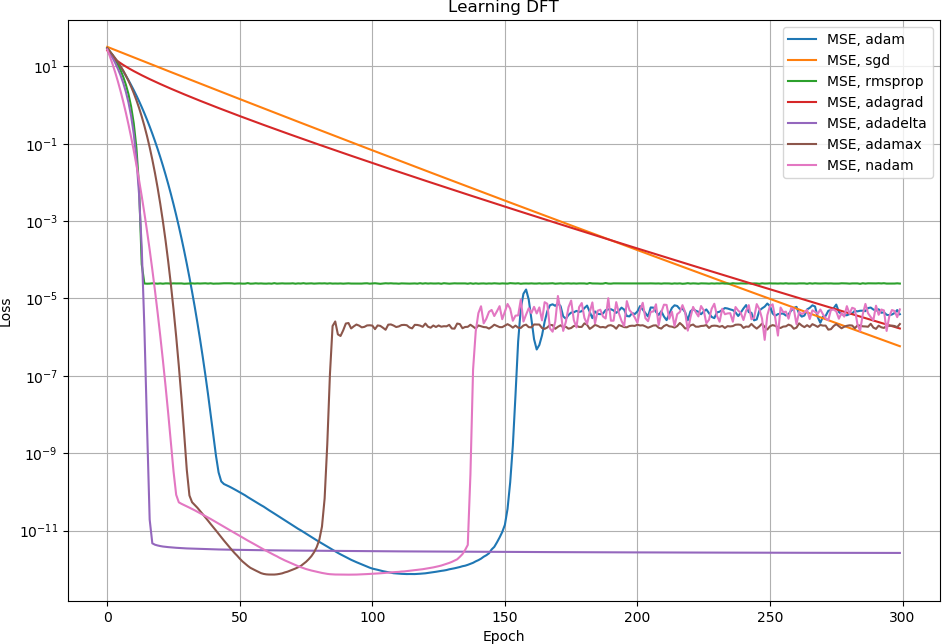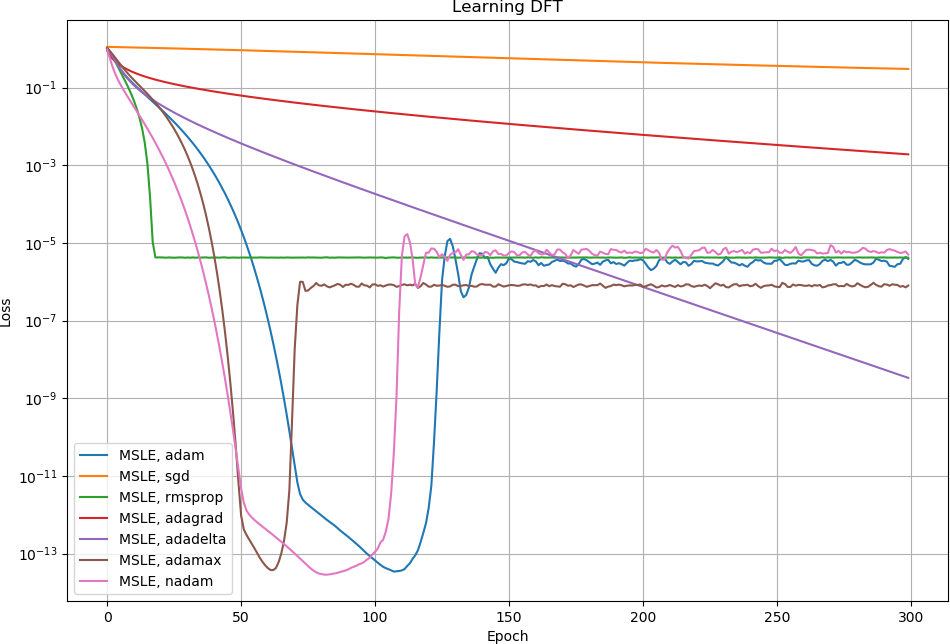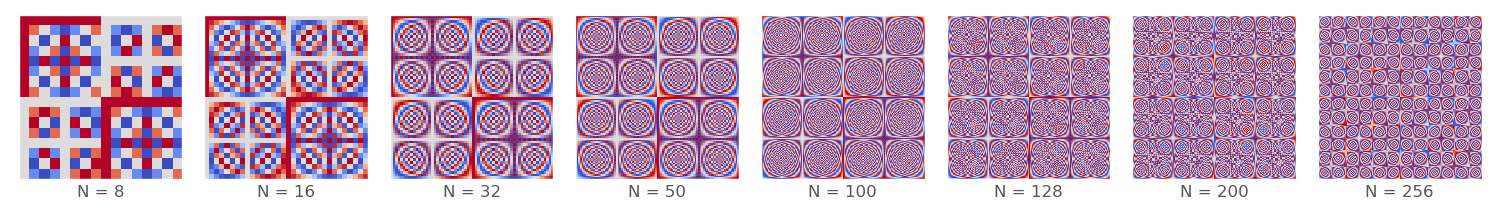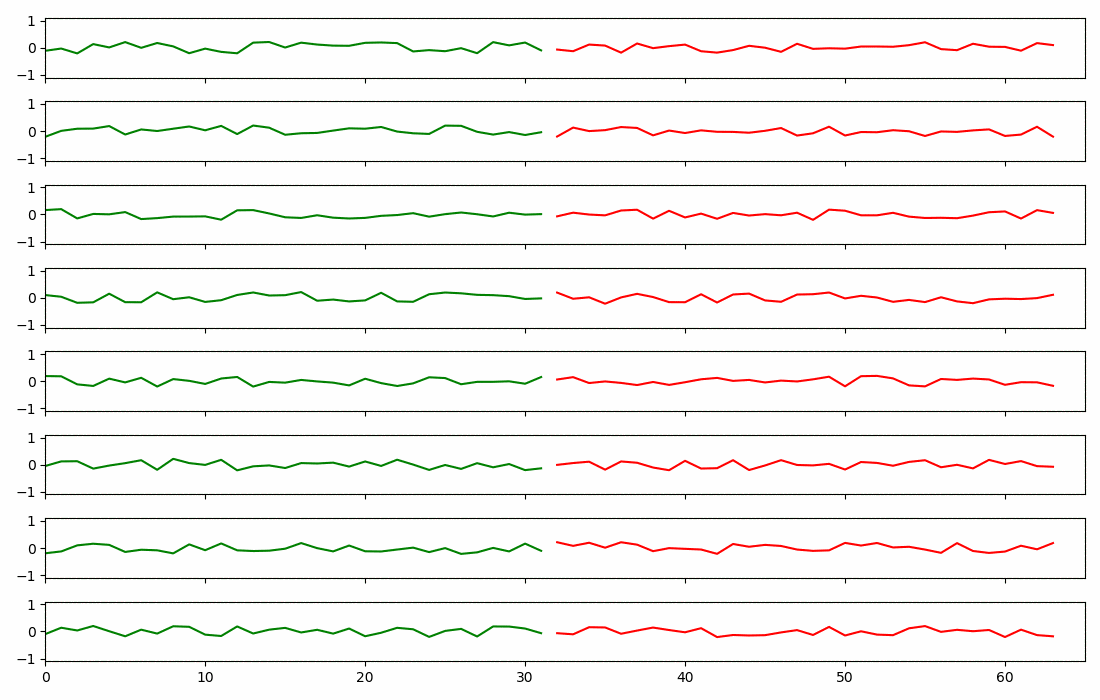{{ message }}

Instantly share code, notes, and snippets.

# endolith/DFT_ANN.py

Last active Jul 15, 2021
Training neural network to implement discrete Fourier transform (DFT/FFT)

My second neural network experiment (first was FIR filter). DFT output is just a linear combination of inputs, so it should be implementable by a single layer with no activation function.Animation of weights being trained:Red are positive, blue are negative. The black squares (2336 out of 4096) are unused, and could be pruned out to save computation time (if I knew how to do that).

Even with pruning, it would be less efficient than an FFT, so if the FFT output is useful, probably best to do it externally and provide it as separate inputs?

This at least demonstrates that neural networks can figure out frequency content on their own, though, if it's useful to the problem.

The loss goes down for a while but then goes up. I don't know why:""" Train a neural network to implement the discrete Fourier transform """ from keras.models import Sequential from keras.layers import Dense import numpy as np import matplotlib.pyplot as plt N = 32 batch = 10000 # Generate random input data and desired output data sig = np.random.randn(batch, N) + 1j*np.random.randn(batch, N) F = np.fft.fft(sig, axis=-1) # First half of inputs/outputs is real part, second half is imaginary part X = np.hstack([sig.real, sig.imag]) Y = np.hstack([F.real, F.imag]) # Create model with no hidden layers, same number of outputs as inputs. # No bias needed. No activation function, since DFT is linear. model = Sequential([Dense(N*2, input_dim=N*2, use_bias=False)]) model.compile(loss='mean_squared_error', optimizer='adam') model.fit(X, Y, epochs=100, batch_size=100) # Confirm that it works data = np.arange(N) def ANN_DFT(x): if len(x) != N: raise ValueError(f'Input must be length {N}') pred = model.predict(np.hstack([x.real, x.imag])[np.newaxis]) result = pred[:N] + 1j*pred[N:] return result ANN = ANN_DFT(data) FFT = np.fft.fft(data) print(f'ANN matches FFT: {np.allclose(ANN, FFT)}') # Heat map of neuron weights plt.imshow(model.get_weights(), vmin=-1, vmax=1, cmap='coolwarm')

###aharchaoumehdi commented Aug 18, 2018

 Have you considered the 2D DFT? I'm wondering if the same network (with higher capacity) would still work.

###endolith commented Aug 18, 2018

 @aharchaoumehdi Yes that would work fine, it would just be a lot of connections and inefficient compared to FFT.

###endolith commented Aug 19, 2018 • edited

 I tested all the optimizers. Some went down and then up, but others just trended downwards and then stopped. Need to learn more about them to understand why.###CYHSM commented Feb 8, 2019

 Thanks for this very nice Gist! The increase in loss after around 100 epochs may come from floating point error accumulation. However I am still surprised by the pattern of the weights, any idea why they look like that? I just ran it again for different N and the pattern stays very similar.###endolith commented Feb 9, 2019 • edited

 @CYHSM Floating point error accumulation in... what? The pattern of weights is just what a DFT does, they're sinusoids of different frequency, discretely, evenly spaced around the unit circle. if you look at a single row or column, it's a sinusoid.

###KasperJuunge commented Oct 21, 2019

 This repo is so cool! I cited it in my thesis 🤓 🙏 👏

###endolith commented Oct 21, 2019

 @KasperJuunge I'd like to see that :D

###KasperJuunge commented Oct 22, 2019

 @KasperJuunge I'd like to see that :D I'll send it when it's done 🤘

###decanbay commented Oct 24, 2019

 maybe it helps to someone https://github.com/decanbay/Fourier-ADALINE

###endolith commented Oct 26, 2019

 @CYHSM Here's a side view of some of the rows:###technolojin commented Aug 12, 2020

 A quick solution would be `sig = sig * 0.01`. Other option is to adjust a parameter 'beta_2'. `adam = tf.keras.optimizers.Adam(learning_rate=0.001, beta_1=0.9, beta_2=0.9998, epsilon=1e-07, amsgrad=False)` `model.compile(loss='mean_squared_error', optimizer=adam)`

###endolith commented Aug 13, 2020

 @technolojin solution to what?

###technolojin commented Aug 13, 2020

 @technolojin solution to what? For the rise of the loss.

###endolith commented Aug 13, 2020

 @technolojin Oh ok, it's more that I don't understand why it happens, though. This was just a learning exercise

###technolojin commented Aug 13, 2020

 @endolith I could learn this basic idea. I would like to thank you. This work inspired me to find another related studies.

###masip85 commented Sep 9, 2020

 I guess this DNN execution is not faster than original fft? Isn't it?

###endolith commented Sep 9, 2020 • edited

 @masip85 No, not at all. It's inaccurate and highly inefficient, and you need to train it on white data if you want it to be correct at all points of the spectrum. It was just an experiment. I'm a signal processing engineer and was trying to learn the basics of ANNs. If you think that your neural network would benefit from FFT features, then I would suggest calculating the FFT and feeding it to the network separately. I also tested if it could learn the absolute value of the FFT output and it did not do very well with ReLUs, they were not good at approximating the absolute value function. Again, I would feed that information directly to the network if I thought it would benefit from it.

###rajb245 commented Sep 21, 2020

 Very nice experiment. For people interested in ML for "fast transforms" of this type, let me leave a link to this work here: https://dawn.cs.stanford.edu/2019/06/13/butterfly/ In the arXiv paper, they show that you can indeed learn fast-transforms that get to the same order of efficiency as a hand-tuned fast implementation. And the results go beyond that, implying that all matrices admit a representation as a composition of only sparse butterfly matrices and permutations.

###endolith commented Sep 21, 2020

 @rajb245 So are they replacing the dense connection layer with a butterfly connection layer?

###rajb245 commented Sep 21, 2020

 @endolith That's one idea they explored in the paper, yes. They showed you can learn some underlying sparse structure of otherwise "fully connected" layers using their method. Using it like that is a new kind of matrix/model compression, and it contrasts a lot with the other approach that's popular in DL, pruning. They also use the ideas of learning the weights of matrices with butterfly-sparsity-patterns and learning a permutation out a discrete set of choices to show that Adam does indeed find solutions that are the usual "fast" linear transforms from signal processing (FFT, fast DCT, fast DST, etc.).

###msaad1311 commented Nov 18, 2020

 Hello. I tried to use your code and tried to find the difference between in computing the FFT using numpy and neural network and there was a big difference. Can you provide some insights on how to solve that? I saw multiple papers that are being published in which the authors mention that using the neural network as FFT reduces the time complexity greatly.

###endolith commented Nov 19, 2020 • edited

 @msaad1311 Yes, it's highly inefficient, as I said in the description and the comments. Even more so than a direct DFT because of all the zero weights being calculated unnecessarily. This isn't something that you should be doing. It was just an experiment while teaching myself neural nets.  If your neural net would benefit from frequency domain information, it's better to just do a numpy FFT and pass the output to the net (possibly adding a magnitude function afterward, since that nonlinearity was much harder to learn in my tests). If you need the net to learn FFT-like transforms in general, look at the comment above about butterfly networks https://gist.github.com/endolith/98863221204541bf017b6cae71cb0a89#gistcomment-3461984

###sebraun-msr commented Jul 8, 2021

 Can you shed some light on the motivation on this? Why do you want to approximate an existing algorithm with a (likely less accurate and less efficient) black box approach?

###endolith commented Jul 8, 2021

 @sebraun-msr It was just an experiment. I thought that ANN nodes could be equivalent to signal processing nodes and tried implementing some things that way. I've said multiple times that you shouldn't actually do this. :) For example, I learned that ANNs can learn linear functions like this very easily, that they only learn at frequencies that are in the dataset (so need to be trained on white noise), tried all the different optimizers, and (in a later experiment) learned that `abs(fft(x))` is much harder to learn with any accuracy: https://stats.stackexchange.com/q/363352/11633 https://stats.stackexchange.com/q/379884/11633